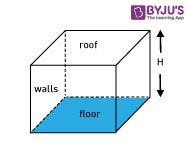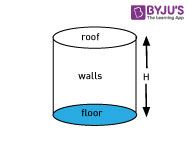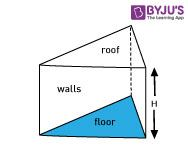# Area Of prism

Prism Definition

A Prism is a solid figure with a uniform cross-section. Here are some examples of Prisms:

Rectangular based PrismCircular-based Prism (Cylinder)Triangular Based PrismSurface Area of Prism

The surface area of a prism is always equal to the sum of the areas of all its faces, which includes the floor, walls, and roof. Because in a prism, the roof and the floor have the same shape and their surface areas are always the same which can be found out by

The surface area of Prism = 2 × Area of the base + Perimeter of the base × Height

The actual formula to find out the circumference is used depending upon the shape of the base

Rectangular PrismBase Shape: Rectangle with length L and with width W

Area of the base: L*W

Perimeter: 2(L+W)

Surface area= 2LW+2(L+W)H

Circular-based Prism (Cylinder)Base Shape: Circle with a radius of R

Area of the Base: PR2

The perimeter of the base: 2PR

Surface area= 2PR2 + 2PRH

Triangular based PrismBase Shape: Triangle with base B, height H and sides S1, S2 and S3

Area of the base: ½ B*H

Perimeter of the base: S1 + S2 +S3

Surface area = BH + (S1+S2+S3)H

To know more about surface area of a prism formula, you can visit us at byjus.com

Q1

### What is Prism?

The prism shape in maths can be defined as a solid figure with a uniform cross-section.

Q2

### What is Meant by the Area of a Prism?

The area of a prism is defined as the total amount of space that the prism encloses in a three-dimensional space.

Q3

### What is the Formula for Surface Area of a Prism?

The surface area of a prism is the summation of twice the area of the base and the product of the perimeter of the base and height. So,

The surface area of Prism = 2 × area of the base + perimeter of the base × height

Q4

### What is the Surface Area of a Rectangular Prism?

The surface area of a rectangular prism is:

A = 2(lb+lh+bh)

Test your Knowledge on Area of Prism